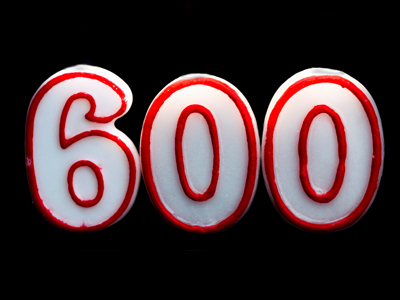The nearest 100 to 641 is 600.

# Rounding Numbers (Year 3/4)

Learning about rounding numbers is a big part of KS2 Maths! In Years Three and Four, kids discover how to round whole numbers to the nearest ten or hundred.

Rounding means making numbers simpler, closer to the nearest ten or hundred. It's like deciding which group of ten or hundred a number belongs to. But, if a number ends in 5 or 50, we always round it up!

Rounded numbers aren't exact, but they're really helpful for big numbers. Try a quiz to see how well you know rounding numbers. Perfect for 7-9 year olds!

1.
Which of these numbers would be rounded down to the nearest 100?
134
250
879
191
Just as 5 is rounded up to 10, 50 is rounded up to 100 so 250 would not be rounded down
2.
Which is the nearest 10 to 33?
10
20
30
40
33 is closer to 30 than 40
3.
What is the nearest 100 to 641?
600
700
800
1,000
As 41 is less than 50, 641 is rounded down to 600
4.
In which of these questions would you need to round the answer up?
A box holds 6 cakes. How many boxes will I need to hold 34 cakes?
Tickets cost £5. I have £22 how many tickets can I buy?
Sweets cost 20p. I have £1. How many sweets can I buy?
A pencil is 10cm. How long will 3 pencils be?
34 ÷ 6 = 5 with 4 left over. As the remaining 4 need to go in a box 6 boxes are needed
5.
Why is rounding 45 more difficult?
It is half way between 40 and 50
It is a multiple of 9
It is an odd number
It is half way between 0 and 50
As 45 is half way between 40 and 50 the rule is to always round 5 up
6.
Which is the nearest 10 to 87?
80
90
100
200
87 is only 3 less than 90
7.
What is the nearest 100 to 850?
700
800
900
1,000
As the number ends in 50 we always round up to the nearest 100
8.
Which is the nearest 100 to 379?
100
200
300
400
379 is closer to 400 than to 300
9.
Which is the nearest 10 to 75?
70
80
90
100
Remember that 5s are rounded up not down
10.
Which of these numbers would be rounded down to the nearest 10?
36
25
19
91
25 is rounded up as it ends in a 5